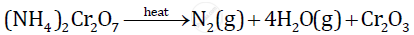Share

# Consider the Following Reaction and Based on the Reaction Answer the Questions that Follow: the Quantity in Moles of (Nh4)2cr2o7 If 63gm Of(Nh4)2cr2o7 is Heated. and the Quantity in Moles of Nitrogen Formed. and the Volume in Liters Or Dm3 of N2 Evolved at S.T.P. and He Mass in Grams of Cr2o3 Formed at the Same Time - ICSE Class 10 - Chemistry

ConceptDeduction of Simple (Empirical) and Molecular Formula from the Percentage Composition of a Compound

#### Question

Consider the following reaction and based on the reaction answer the questions that follow:Calculate:

1) the quantity in moles of (NH4)2Cr2O7 if 63gm of(NH4)2Cr2O7 is heated.

2) the quantity in moles of nitrogen formed.

3) the volume in liters or dm3 of N2 evolved at S.T.P.

4) the mass in grams of Cr2O3 formed at the same time

(Atomic masses: H=1, Cr= 52, N=14]

#### Solution

The given reaction is as follows:1) Given:

Weight of (NH4)2Cr2O7 = 63 gm

Molar mass of (NH4)2Cr2O7

= (2 x 14) + (8 x 1) + (2 x 52) + (7 x 16)

= 28 + 8 + 104 + 112

= 252 gm

1 mole (NH4)2Cr2O7 = 252 gm

Hence, 63 gm of (NH4)2Cr2O7 = 0.25 moles

The quantity of moles of (NH4)2Cr2O7 if 63 gm of (NH4)2Cr2O7 is heated is 0.25 moles.

2) From the given chemical equation, 1 mole of (NH4)2Cr2O7 produces 1 mole of nitrogen gas.

Hence, 0.25 moles of (NH4)2Cr2O7 can produce 0.25 moles of nitrogen gas.

The quantity in moles of nitrogen formed is 0.25 moles

3) One mole of an ideal gas at S.T.P. occupies 22.4 liters or dm3.

Hence, 0.25 moles of (NH4)2Cr2O7 will occupy 0.25  22.4 = 5.6 litres or dm3

The volume in liters or dm3 of N2 evolved at S.T.P. is 5.6 liters or dm3.

4)From the given chemical equation, 1 mole of (NH4)2Cr2O7 produces 1 mole of Cr2O3.

Hence, 0.25 moles of (NH4)2Cr2O7 will produce 0.25 moles of Cr2O3

Molar mass of Cr2O3

= (2 x 52) + (3 x 16)

= 104 + 48

= 152 gm

1 mole Cr2O= 152 gm

Hence, 0.25 moles of Cr2O3 = 0.25  152 = 38 gm

The mass in grams of Cr2O3 formed at the same time is 38 gm.

Is there an error in this question or solution?

#### APPEARS IN

Solution Consider the Following Reaction and Based on the Reaction Answer the Questions that Follow: the Quantity in Moles of (Nh4)2cr2o7 If 63gm Of(Nh4)2cr2o7 is Heated. and the Quantity in Moles of Nitrogen Formed. and the Volume in Liters Or Dm3 of N2 Evolved at S.T.P. and He Mass in Grams of Cr2o3 Formed at the Same Time Concept: Deduction of Simple (Empirical) and Molecular Formula from the Percentage Composition of a Compound.
S